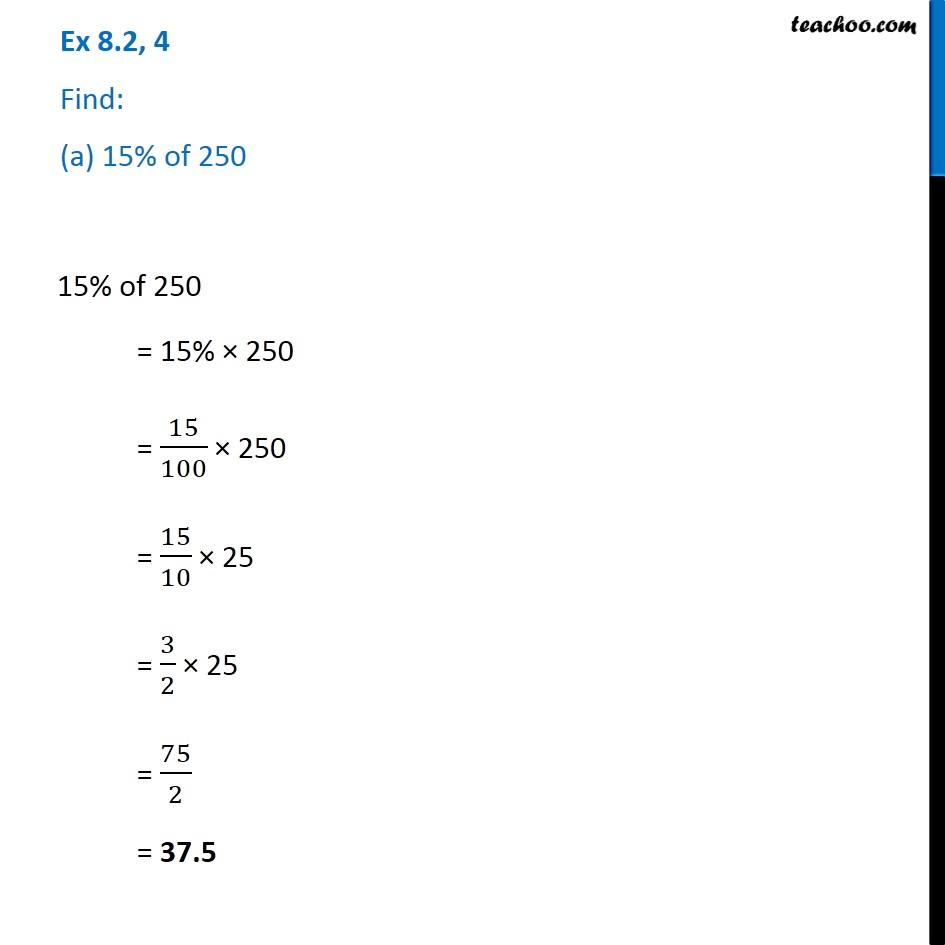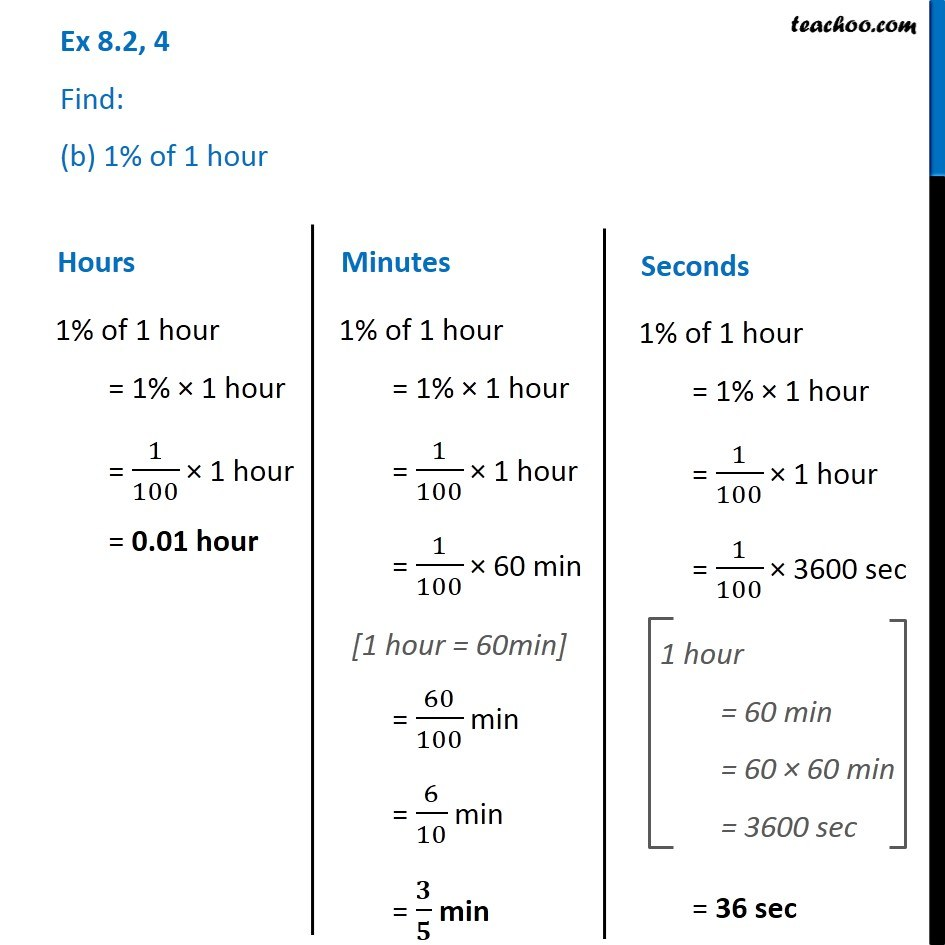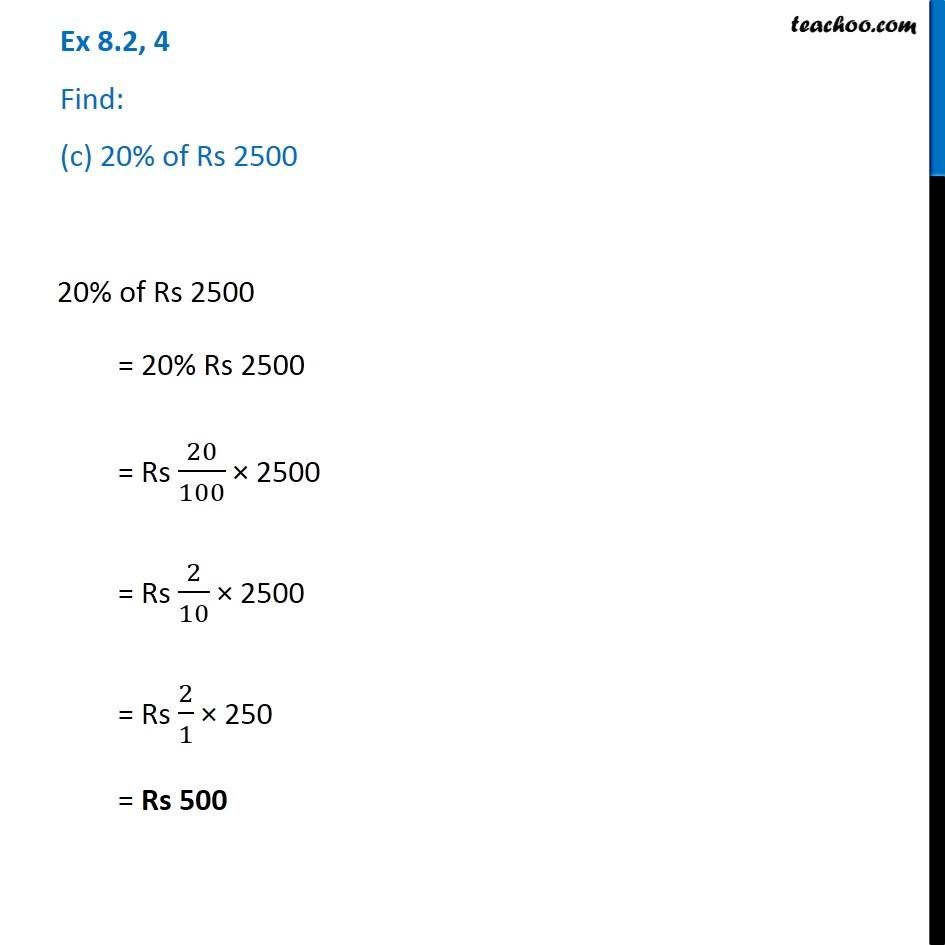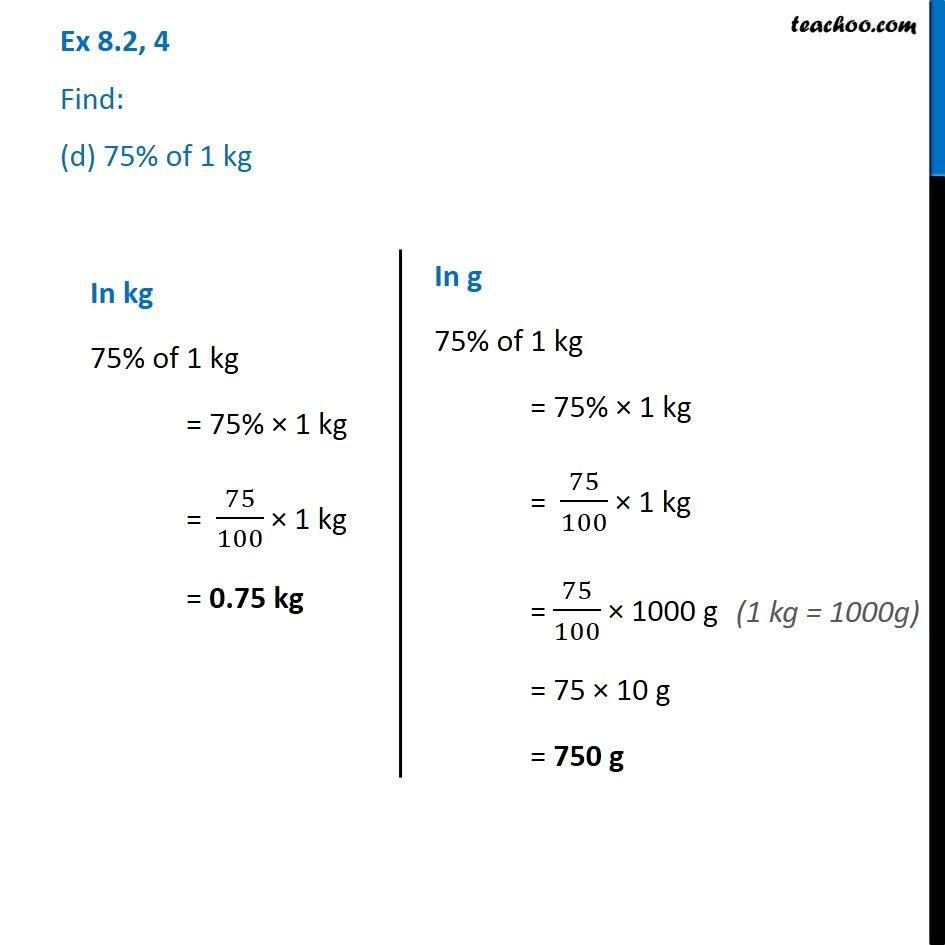1. Chapter 8 Class 7 Comparing Quantities
2. Serial order wise
3. Ex 8.2

Transcript

Ex 8.2, 4 Find: (a) 15% of 250 15% of 250 = 15% × 250 = 15/100 × 250 = 15/10 × 25 = 3/2 × 25 = 75/2 = 37.5 Ex 8.2, 4 Find: (b) 1% of 1 hour Hours 1% of 1 hour = 1% × 1 hour = 1/100 × 1 hour = 0.01 hour 1% of 1 hour = 1% × 1 hour = 1/100 × 1 hour = 1/100 × 60 min = 60/100 min = 6/10 min = 𝟑/𝟓 min 1% of 1 hour = 1% × 1 hour = 1/100 × 1 hour = 1/100 × 3600 sec = 36 sec 1 hour = 60 min = 60 × 60 min = 3600 sec Ex 8.2, 4 Find: (c) 20% of Rs 2500 20% of Rs 2500 = 20% Rs 2500 = Rs 20/100 × 2500 = Rs 2/10 × 2500 = Rs 2/1 × 250 = Rs 500 Ex 8.2, 4 Find: (d) 75% of 1 kg In kg 75% of 1 kg = 75% × 1 kg = 75/100 × 1 kg = 0.75 kg In g 75% of 1 kg = 75% × 1 kg = 75/100 × 1 kg = 75/100 × 1000 g = 75 × 10 g = 750 g (1 kg = 1000g)

Ex 8.2